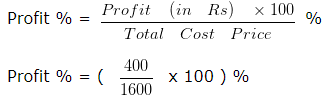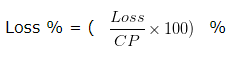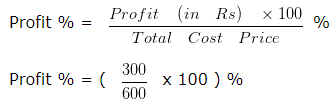# Profit and Loss Worksheet for Grade 5Q.1) Mr Gupta bought some goods for ₹ 200 and sold it for ₹ 350 . What was his Profit ?
a) ₹ 100
b) ₹ 300
c) ₹ 150

Q.2) A girl bought goods worth ₹ 900 . Her overhead expenses were ₹ 225 . She sold the goods for ₹ 2000 . What was her Profit ?
a) ₹ 875
b) ₹ 825
c) ₹ 1075

Q.3) If Cost Price = ₹ 250 , Overhead expenses = ₹ 52 and Selling Price = ₹ 140 . Find the loss ?
a) Loss = ₹ 168
b) Loss = ₹ 162
c) Loss = ₹ 164

Q.4) Mohit bought a Decorative lamp for ₹ 1500 and spent ₹ 100 on transportation . He sold the Decorative lamp for ₹ 2000 . Find His profit percent ?
a) Profit % = 25 %
b) Profit % = 30 %
c) Profit % = 28 %

Q.5) If Cost Price = ₹ 95 , Overhead expenses = ₹ 21 and Selling Price = ₹ 180 . Find the Profit ?
a) Profit = ₹ 64
b) Profit = ₹ 66
c) Profit = ₹ 59

Q.6) Find the Cost Price, If the Selling Price is ₹ 394 and the profit is ₹ 116 ?
a) ₹ 288
b) ₹ 278
c) ₹ 284

Q.7) Mahendra bought a Printer for ₹ 3000 and sold it for ₹ 2880 . Find his loss percent ?
a) Loss % = 4 %
b) Loss % = 10 %
c) Loss % = 7 %

Q.8) If a school bag is bought for ₹ 600 and sold for ₹ 900 . What is the profit percent ?
a) Profit % = 45 %
b) Profit % = 50 %
c) Profit % = 40 %

Q.9) Find the Cost Price If the Selling Price is ₹ 140 and the loss is ₹ 35 ?
a) ₹ 171
b) ₹ 175
c) ₹ 164

Q.10) Find the Selling Price If the Cost Price is ₹ 240 and the loss is ₹ 63 ?
a) ₹ 177
b) ₹ 169
c) ₹ 174

Q.11) Find the Selling Price . If the Cost Price is ₹ 110 and the profit is ₹ 22 ?
a) ₹ 132
b) ₹ 140
c) ₹ 127

Q.12) If Cost Price = ₹ 225 and Selling Price = ₹ 200 . Find the Loss ?
a) Loss = ₹ 20
b) Loss = ₹ 17
c) Loss = ₹ 25

### Profit and Loss Worksheet for Grade 5 Explanations

Q.1) Explanation – Profit and Loss Worksheet for Grade 5

Cost Price of goods = ₹ 200
Selling Price of goods = ₹ 350
Cost Price (C.P) = Price at which an Article is purchased.
Selling Price (S.P) = Price at which the Article is sold.
Since, Selling Price > Cost Price , Mr Gupta made a profit
Profit = Selling Price – Cost Price
Profit = 350 – 200
= 150
So, Profit made by Mr Gupta is ₹ 150

Correct Answer – c) ₹ 150

Q.2) Explanation – Profit and Loss Worksheet for Grade 5

Cost Price of goods = ₹ 900
Selling Price of goods = ₹ 2000
Cost Price ( CP ) = Price at which an Article is purchased (including overhead).
Selling Price ( SP ) = Price at which the Article is sold.
Overhead expenses = Overhead expenses are certain other expenses incurred by the Seller, and hence are added to his cost price.
Therefore,
Total Cost Price = Cost Price + Overhead expenses
Total Cost Price = 900 + 225
Total Cost Price = ₹ 1125
Since,
Total Cost Price < Selling Price , this indicates that there is a profit
Therefore,
Profit = Selling Price – Total Cost Price
Profit = 2000 – 1125
= 875
Profit = ₹ 875
So, the profit made by the girl is ₹ 875

Correct Answer – a) ₹ 875

Q.3) Explanation – Profit and Loss Worksheet for Grade 5

Cost Price = ₹ 250
Selling Price = ₹ 140
Cost Price (C.P) = Price at which an Article is purchased (including overhead expenses)
Selling Price (S.P) = Price at which the Article is sold.
Overhead expenses = Overhead expenses are certain other expenses incurred by the Seller, and hence are added to his cost price.
Therefore,
Total Cost Price = Cost Price + Overhead expenses
Total Cost Price = 250 + 52
Total Cost Price = ₹ 302
Since, Total Cost Price > Selling Price
Therefore,
Loss = Total Cost Price – Selling Price
Loss = 302 – 140
So, Loss = ₹ 162

Correct Answer – b) Loss = ₹ 162

Q.4) Explanation – Profit and Loss Worksheet for Grade 5

Cost Price of Decorative lamp = ₹ 1500
Transportation charges are = ₹ 100
Transportation charge is an overhead expenses
Overhead expenses = Overhead expenses are certain other expenses incurred by the Seller, and hence are added to his cost price.
Therefore,
Total Cost Price = Cost Price + Overhead expenses
Total Cost Price = 1500 + 100
Total Cost Price = ₹ 1600
Selling Price of Decorative lamp = ₹ 2000
Clearly, Selling Price > Total Cost Price
This indicates some profit has been earned
Therefore,
Profit = Selling Price – Total Cost Price
Profit = 2000 – 1600
Profit = ₹ 400
Since,Profit % = 25 %
So, the Profit % is 25 %

Correct Answer – a) Profit % = 25 %

Q.5) Explanation – Profit and Loss Worksheet for Grade 5

Cost Price = ₹ 95
Selling Price = ₹ 180
Cost Price (C.P) = Price at which an Article is purchased.
Selling Price (S.P) = Price at which the Article is sold.
Overhead expenses = Overhead expenses are certain other expenses incurred by the Seller, and hence are added to his cost price.
Therefore,
Total Cost Price = Cost Price + Overhead expenses
Total Cost Price = 95 + 21
Total Cost Price = ₹ 116
Since, Selling Price > Total Cost Price
Therefore,
Profit = Selling Price – Total Cost Price
Profit = 180 – 116
Profit = 64
So, Profit = ₹ 64

Correct Answer – a) Profit = ₹ 64

Q.6) Explanation – Profit and Loss Worksheet for Grade 5

Selling Price = ₹ 394
Profit = ₹ 116
Cost Price = ?
When profit is earned, then Cost Price would be less than the Selling Price
Therefore,
Profit = Selling Price – Cost Price
Or we can say,
Cost Price = Selling Price – Profit
Cost Price = 394 – 116
Cost Price = 278
So, Cost Price = ₹ 278

Correct Answer – b) ₹ 278

Q.7) Explanation – Profit and Loss Worksheet for Grade 5
Cost price(CP) – Price at which an article is purchased.
Selling price(SP) – Price at which an article is sold.
Loss – Where the Cost Price is greater than the Selling Price, the difference between the SP and CP is the LossCost Price = ₹ 3000
Selling Price = ₹ 2880
Clearly, Cost Price > Selling Price
In this case a loss has been incurred
We know that,
Loss = Cost Price – Selling Price
Loss = 3000 – 2880
Loss = ₹ 120
Therefore,
Loss % = [(120/3000) x 100 ] %
Loss % = 4 %
So, the Loss % is 4 %

Correct Answer – a) Loss % = 4 %

Q.8) Explanation – Profit and Loss Worksheet for Grade 5
Cost Price of school bag = ₹ 600
Selling Price of school bag = ₹ 900
Cost Price (CP) – Price at which an Article is purchased.
Selling Price (SP) – Price at which the Article is sold.
Profit – Where the Selling Price is greater than the Cost Price, the difference between the SP and CP is the gain
Clearly,
Selling Price > Cost Price
It means a profit has been earned
Since,
Profit = Selling Price – Cost Price
Profit = 900 – 600
Profit = ₹ 300
Now, we have to calculate profit percent= 50 %
So, the Profit % is 50 %

Correct Answer – b) Profit % = 50 %

Q.9) Explanation – Profit and Loss Worksheet for Grade 5
Selling Price = ₹ 140
Loss = ₹ 35
Cost Price = ?
When loss is suffered, , the Cost Price would be more than Selling Price
Therefore,
Loss = Cost Price – Selling Price
Or we can say,
Cost Price = Selling Price + Loss
Cost Price = 140 + 35
Cost Price = 175
So, Cost Price = ₹ 175

Correct Answer – b) ₹ 175

Q.10) Explanation – Profit and Loss Worksheet for Grade 5
Cost Price = ₹ 240
Loss = ₹ 63
Selling Price = ?
When the loss is suffered by Seller, then the Selling Price would be less than the Cost Price
Therefore,
Loss = Cost Price – Selling Price
Or we can say,
Selling Price = Cost Price – Loss
Selling Price = 240 – 63
= 177
So, Selling Price = ₹ 177

Correct Answer – a) ₹ 177

Q.11) Explanation – Profit and Loss Worksheet for Grade 5
Cost Price = ₹ 110
Profit = ₹ 22
Selling Price = ?
When profit is earned, then the selling price would be more than the cost price
Therefore,
Profit = Selling Price – Cost Price
Or we can say,
Selling Price = Cost Price + Profit
Selling Price = 110 + 22
Selling Price = 132
So, Selling Price = ₹ 132

Correct Answer – a) ₹ 132

Q.12) Explanation – Profit and Loss Worksheet for Grade 5
Cost Price = ₹ 225
Selling Price = ₹ 200
Cost Price ( CP ) = Price at which an Article is purchased.
Selling Price ( SP ) = Price at which the Article is sold.
Since, Cost Price > Selling Price this indicates there is a loss
Therefore,
Loss = Cost Price – Selling Price
Loss = 225 – 200
= 25
So, Loss = ₹ 25

Correct Answer – c) Loss = ₹ 25